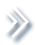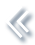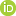####ArchivesReview Articles

## Efficient Robbins–Monro procedure for multivariate binary data

Cui Xiong ,

School of Statistics, East China Normal University, Shanghai, People's Republic of ChinaJin Xu

School of Statistics, East China Normal University, Shanghai, People's Republic of Chinajxu@stat.ecnu.edu.cn

Pages 172-180 | Received 06 Jan. 2018, Accepted 31 Jul. 2018, Published online: 07 Aug. 2018,
• Abstract
• References
• Citations

Abstract

This paper considers the problem of jointly estimating marginal quantiles of a multivariate distribution. A sufficient condition for an estimator that converges in probability under a multivariate version of Robbins–Monro procedure is provided. We propose an efficient procedure which incorporates the correlation structure of the multivariate distribution to improve the estimation especially for cases involving extreme marginal quantiles. Estimation efficiency of the proposed method is demonstrated by simulation in comparison with a general multivariate Robbins–Monro procedure and an efficient Robbins–Monro procedure that estimates the marginal quantiles separately.

## References

1. Anbar, D. (1978). A stochastic Newton–Raphson method. Journal of Statistical Planning and Inference2, 153–163. doi: 10.1016/0378-3758(78)90004-6 [Google Scholar]
2. Azzalini, A. (2014). The skew-normal and related families. Cambridge: Cambridge University Press. [Google Scholar]
3. Azzalini, A., & Capitanio, A. (1999). Statistical applications of the multivariate skew normal distribution. Journal of the Royal Statistical Society, Series B61, 579–602. doi: 10.1111/1467-9868.00194 [Google Scholar]
4. Chaloner, K., & Larntz, K. (1989). Optimal Bayesian design applied to logistic regression experiments. Journal of Statistical Planning and Inference21, 191–208. doi: 10.1016/0378-3758(89)90004-9 [Google Scholar]
5. Chaudhuri, P., & Mykland, P. A. (1993). Nonlinear experiments: Optimal design and inference based on likelihood. Journal of the American Statistical Association88, 538–546. doi: 10.1080/01621459.1993.10476305 [Taylor & Francis Online], [Google Scholar]
6. Cheung, Y. K. (2010). Stochastic approximation and modern model-based designs for dose-finding clinical trials. Statistical Science25, 191–201. doi: 10.1214/10-STS334 [Google Scholar]
7. Chung, K. L. (1954). On a stochastic approximation method. Annals of Mathematical Statistics25, 463–483. doi: 10.1214/aoms/1177728716 [Google Scholar]
8. Dror, H. A., & Steinberg, D. M. (2006). Robust experimental design for multivariate generalized linear models. Technometrics48, 520–529. doi: 10.1198/004017006000000318 [Taylor & Francis Online], [Google Scholar]
9. Dror, H. A., & Steinberg, D. M. (2008). Sequential experimental designs for generalized linear models. Journal of the American Statistical Association103, 288–298. doi: 10.1198/016214507000001346 [Taylor & Francis Online], [Google Scholar]
10. Duflo, M. (1997). Random iterative models. Berlin: Springer-Verlag. [Google Scholar]
11. Hung, Y., & Joseph, V. R. (2014). Discussion of “Three-phase optimal design of sensitivity experiments” by Wu and Tian. Journal of Statistical Planning and Inference149, 16–19. doi: 10.1016/j.jspi.2013.12.011 [Google Scholar]
12. Joseph, V. R. (2004). Efficient Robbins–Monro procedure for binary data. Biometrika91, 461–470. doi: 10.1093/biomet/91.2.461 [Google Scholar]
13. Kotz, S., & Nadarajah, S. (2004). Multivariate t distributions and their applications. Cambridge: Cambridge University Press. [Google Scholar]
14. Lai, T. L., & Robbins, H. (1979). Adaptive design and stochastic approximation. Annals of Statistics7, 1196–1221. doi: 10.1214/aos/1176344840 [Google Scholar]
15. Neyer, B. T. (1994). A D-optimality-based sensitivity test. Technometrics36, 61–70. doi: 10.2307/1269199 [Google Scholar]
16. Robbins, H., & Monro, S. (1951). A stochastic approximation method. Annals of Mathematical Statistics22, 400–407. doi: 10.1214/aoms/1177729586 [Google Scholar]
17. Ruppert, D. (1985). A Newton-Raphson version of the multivariate Robbins-Monro procedure. Annals of Statistics13, 236–245. doi: 10.1214/aos/1176346589 [Google Scholar]
18. Ruppert, D. (1988). Efficient estimators from a slowly convergent Robbins–Monro process. School of Operations Research and Industrial Engineering Technical Report, 781. Cornell University, Ithaca, NY. [Google Scholar]
19. Sacks, J. (1958). Asymptotic distribution of stochastic approximation procedures. Annals of Mathematical Statistics29, 373–405. doi: 10.1214/aoms/1177706619 [Google Scholar]
20. Wei, C. Z. (1987). Multivariate adaptive stochastic approximation. Annals of Statistics15, 1115–1130. doi: 10.1214/aos/1176350496 [Google Scholar]
21. Wetherill, G. B. (1963). Sequential estimation of quantal response curves. Journal of the Royal Statistical Society, Series B25, 1–48. [Google Scholar]
22. Wu, C. F. J. (1985). Efficient sequential designs with binary data. Journal of the American Statistical Association80, 974–984. doi: 10.1080/01621459.1985.10478213 [Taylor & Francis Online], [Google Scholar]
23. Wu, C. F. J. (1986). Maximum likelihood recursion and stochastic approximation in sequential designs. In J. V. Ryzin (Ed.), IMS monograph series: Vol. 8. Adaptive statistical procedures and related topics (pp. 298–314). Hayward, CA: Institute of Mathematical Statistics. [Google Scholar]
24. Wu, C. F. J., & Tian, Y. (2014). Three-phase optimal design of sensitivity experiments. Journal of Statistical Planning and Inference149, 1–15. doi: 10.1016/j.jspi.2013.10.007 [Google Scholar]
25. Young, L. J., & Easterling, R. G. (1994). Estimation of extreme quantiles based on sensitivity tests: A comparative study. Technometrics36, 48–60. doi: 10.1080/00401706.1994.10485400 [Taylor & Francis Online], [Google Scholar]